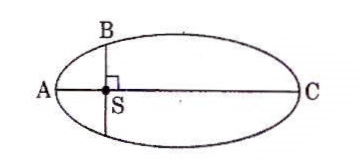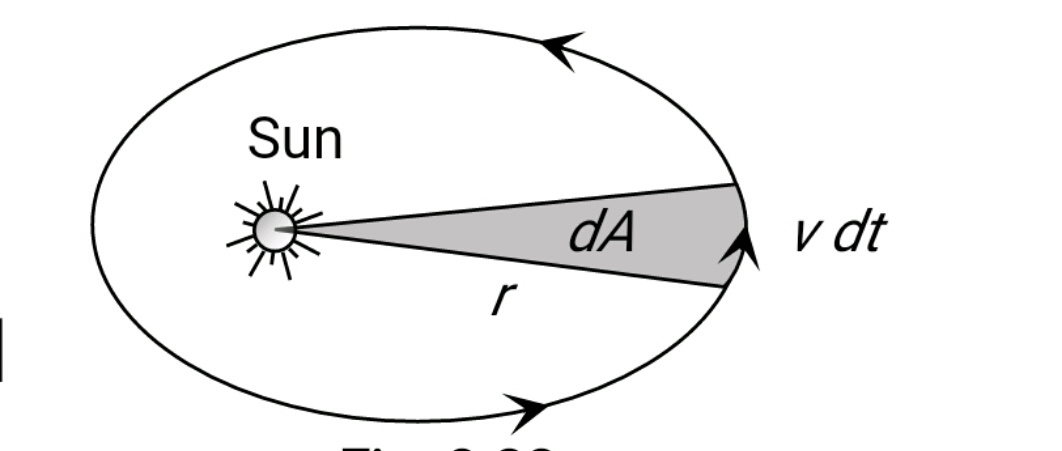Q

# Solve it, The kinetic energies of a planet in an elliptical orbit about the Sun, at positions A, B and C are KA, KB and KC, respectively. AC is the major axis and SB is perpendicular to AC at the position of the Sun S as shown in the figure. Then

The kinetic energies of a planet in an elliptical orbit about the Sun, at positions A, B and C are KA, KB and KC, respectively. AC is the major axis and SB is perpendicular to AC at the position of the Sun S as shown in the figure. Then• Option 1)

• Option 2)

• Option 3)

• Option 4)

977 Views

Option 2

As we have learned

Kepler's 2nd law -

Area of velocity =Areal velocity

small area traced

- wherein

Simiar to Law of conservation of momentum

Angular momentum

Known  as Law of Area

Option 1)

This is incorrect

Option 2)

This is correct

Option 3)

This is incorrect

Option 4)

This is incorrect

Exams
Articles
Questions